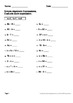Algebra Worksheets\$5.00Package includes 120 worksheets.

Simple Algebraic Expressions (30 Worksheets)
Example:
a = 2
3 + a + 2 = ?

Expressions with 1 variable (30 Worksheets)
Example:
c - 2 = 4
c = ?

Expressions with 2 variables (30 Worksheets)
Example:
b x 3 = a , b = 4
a = ?

Algebraic expressions: True or False? (30 Worksheets)
Example:
a + 2 = 5 , a = 10
True or False?

----------------------------------

You May Also Like These Task Cards: